• 对于游戏行业程序员来说，向量“点乘”叉乘”是非常熟悉的运算。从代码上看他们很简单：（以下代码选自UE4的“Vector.h”） 点乘就是各分量逐项相乘，最终得到了一个标量： FORCEINLINE float FVector::Dot...
目标
对于游戏行业程序员来说，向量“点乘”和“叉乘”是非常熟悉的运算。从代码上看他们运算过程并不复杂：（以下代码选自UE4的“Vector.h”）
点乘就是各分量逐项相乘，最终得到了一个标量：
FORCEINLINE float FVector::DotProduct(const FVector& A, const FVector& B)
{
return X*V.X + Y*V.Y + Z*V.Z;
}

叉乘最终得到一个新的向量，虽然其运算现在看起来略显“奇怪”（不过，在随后的证明中可以看出其重要的几何意义）：
FORCEINLINE FVector FVector::CrossProduct(const FVector& A, const FVector& B)
{
return FVector
(
Y * V.Z - Z * V.Y,
Z * V.X - X * V.Z,
X * V.Y - Y * V.X
);
}

即：
$\vec{a}\cdot\vec{b} = x_ax_b+y_ay_b+z_az_b$
$\vec{a}\times\vec{b} = (y_az_b-z_ay_b,z_ax_b-x_az_b,x_ay_b-y_ax_b)$
他们各自都有重要的几何意义，经常会出现在有关空间计算的逻辑中，例如：

标准化后向量点乘得到的值为夹角的余弦。这样，只要计算点乘，得到-1~ 0 ~1，便可知道两个向量之间方向的关系是相反 ~ 垂直 ~ 相同。
向量叉乘后得到的新向量一定和原先两个向量垂直。
向量叉乘后得到的向量的模，其值为两个向量构成的三角形的面积的二倍。

向量点乘和叉乘的这些特性经常被使用，但是对于其中的数学原理我是模糊不清的，因此我想自己动手证明一下。
而为了让这些证明得更容易，我还需要在此之前证明其基于的一些定理。
1. 证明：勾股定理
定理：
对于一个直角三角形ABC，其中直角为∠BAC，有：
$BC^2=AB^2+AC^2$

其证明网上有很多种，下面的方法据说来自于欧几里得的《几何原本》。（图片来源勾股定理_百度百科）
由于 三角形FBC 和正方形GFBA 同底等高，所以有：
$S_{FBC}\times2=S_{GFBA}$
由于 三角形FBC 和 三角形ABD 为全等三角形，所以有：
$S_{FBC}=S_{ABD}$
由于 三角形ABD 和矩形BDLK 同底等高，所以有：
$S_{ABD}\times2=S_{BDLK}$
因此：
$S_{GFBA}=S_{FBC}\times2=S_{ABD}\times2=S_{BDLK}$
同理：
$S_{ACIH}=S_{KLEC}$
（即图中粉色正方形的面积等于粉色矩形的面积，蓝色正方形的面积等于蓝色矩形的面积）
因此：
\begin{aligned} BC^2 & =S_{BDEC}\\ & =S_{BDLK}+S_{KLEC}\\ & =S_{GFBA}+S_{ACIH}\\ & = AB^2+AC^2\\ \end{aligned}
2. 证明：余弦定理
定理：
对于任意一个三角形ABC，有：
$AB^2=BC^2+AC^2-2BC \cdot AC \cdot cosC$

证明网上也有很多种，下面的方法据说也来自于欧几里得的《几何原本》。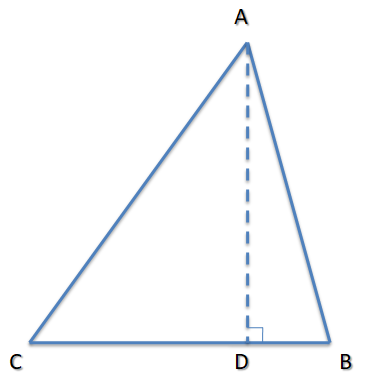$AD = AC \cdot sinC$
$CD = AC \cdot cosC$
在CB边上，有：
$DB= BC-CD=BC- AC \cdot cosC$
\begin{aligned} AB^2 & =AD^2+DB^2\\ & =(AC \cdot sinC)^2+(BC- AC \cdot cosC)^2\\ & =AC^2\cdot sinC^2+BC^2+AC^2\cdot cosC^2-2BC \cdot AC \cdot cosC\\ & =BC^2+AC^2\cdot sinC^2+AC^2\cdot cosC^2-2BC \cdot AC \cdot cosC\\ & =BC^2+AC^2(sinC^2+cosC^2)-2BC \cdot AC \cdot cosC\\ & =BC^2+AC^2-2BC \cdot AC \cdot cosC\\ \end{aligned}
3. 证明：向量点乘的几何意义——结果为模相乘再乘夹角余弦
向量点乘的定义如下：
$\vec{a}\cdot\vec{b} = x_ax_b+y_ay_b+z_az_b$
现在想证明的是：（其中θ为两向量夹角）
$\vec{a}\cdot\vec{b} =\left \| \vec{a} \right \|\cdot\left \| \vec{b} \right \|cos\theta$

在证明前，先看一个算不上是“定理”的结论：
对于一个2维的向量(x,y)，由于坐标系是垂直的，所以由勾股定理很容易能推导出
$2维向量长度^2=x^2+y^2$
对于一个3维的向量(x,y,z)，也可以很容易能推导出：
$3维向量长度^2=x^2+y^2+z^2$

下面正式开始证明：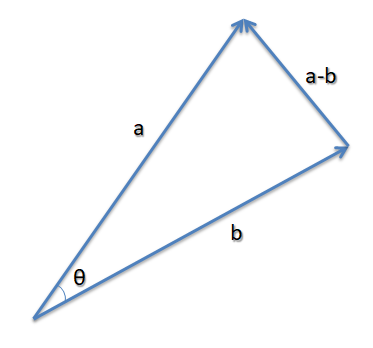如果将向量a和向量b的起点放在一起，那么这两个向量终点之间的向量即为 a-b。
而根据余弦定理，有：
$\left \| \vec{a} -\vec{b} \right \|^2=\left \| \vec{a} \right \|^2 +\left \| \vec{b} \right \|^2 -2\left \| \vec{a} \right \|\cdot\left \| \vec{b} \right \|cos\theta$
写成分量的形式，就是：
$((x_a,y_a,z_a)-(x_b,y_b,z_b))^2=(x_a,y_a,z_a)^2+(x_b,y_b,z_b)^2-2\left \| \vec{a} \right \|\cdot\left \| \vec{b} \right \|cos\theta$
向量相减即各分量相减，即：
$(x_a-x_b,y_a-y_b,z_a-z_b)^2=(x_a,y_a,z_a)^2+(x_b,y_b,z_b)^2-2\left \| \vec{a} \right \|\cdot\left \| \vec{b} \right \|cos\theta$
将平方的运算展开：
$x_a^2+x_b^2-2x_ax_b+y_a^2+y_b^2-2y_ay_b+z_a^2+z_b^2-2z_az_b=x_a^2+x_b^2+y_a^2+y_b^2+z_a^2+z_b^2-2\left \| \vec{a} \right \|\cdot\left \| \vec{b} \right \|cos\theta$
去掉等式两边重复项可得：
$-2x_ax_b-2y_ay_b-2z_az_b=-2\left \| \vec{a} \right \|\cdot\left \| \vec{b} \right \|cos\theta$
约去-2得：
$x_ax_b+y_ay_b+z_az_b=\left \| \vec{a} \right \|\cdot\left \| \vec{b} \right \|cos\theta$
结合向量点乘的定义，则最后可知：
\begin{aligned} \vec{a}\cdot\vec{b} & =x_ax_b+y_ay_b+z_az_b \\ & =\left \| \vec{a} \right \|\cdot\left \| \vec{b} \right \|cos\theta \\ \end{aligned}
4. 证明：向量叉乘的几何意义——结果与原先两个向量都垂直
向量叉乘的定义如下：
$\vec{a}\times\vec{b} = (y_az_b-z_ay_b,z_ax_b-x_az_b,x_ay_b-y_ax_b)$
由前面证明的向量点乘的几何意义可知，
如果能证明：
$(\vec{a}\times\vec{b} )\cdot \vec{a}=0$
$(\vec{a}\times\vec{b} )\cdot \vec{b}=0$
则意味着向量叉乘的结果向量，和原先两个向量的夹角的余弦值都为0，即夹角为90°，即与原先两个向量都垂直

而这个证明可以直接从算式中得出：
\begin{aligned} (\vec{a}\times\vec{b} )\cdot \vec{a} & = (y_az_b-z_ay_b,z_ax_b-x_az_b,x_ay_b-y_ax_b) \cdot (x_a,y_a,z_a) \\ & =y_az_bx_a-z_ay_bx_a+z_ax_by_a-x_az_by_a+x_ay_bz_a-y_ax_bz_a\\ & =(y_az_bx_a-x_az_by_a)+(x_ay_bz_a-z_ay_bx_a)+(z_ax_by_a-y_ax_bz_a)\\ & =0+0+0\\ & =0\\ \end{aligned}
\begin{aligned} (\vec{a}\times\vec{b} )\cdot \vec{b} & = (y_az_b-z_ay_b,z_ax_b-x_az_b,x_ay_b-y_ax_b) \cdot (x_b,y_b,z_b) \\ & =y_az_bx_b-z_ay_bx_b+z_ax_by_b-x_az_by_b+x_ay_bz_b-y_ax_bz_b\\ & =(y_az_bx_b-y_ax_bz_b)+(z_ax_by_b-z_ay_bx_b)+(x_ay_bz_b-x_az_by_b)\\ & =0+0+0\\ & =0\\ \end{aligned}
5. 证明：向量叉乘的几何意义——结果的模为原先两向量的模相乘再乘夹角正弦
向量叉乘的定义如下：
$\vec{a}\times\vec{b} = (y_az_b-z_ay_b,z_ax_b-x_az_b,x_ay_b-y_ax_b)$
现在想证明的是：（其中θ为两向量夹角）
$\left \|\vec{a}\times\vec{b}\right \| =\left \| \vec{a} \right \|\left \| \vec{b} \right \|sin\theta$

（方法来自于《3D游戏与计算机图形学中的数学方法》）
取向量叉乘结果的平方，逐步展开：
\begin{aligned} \left \|\vec{a}\times\vec{b}\right \| ^2 & = (y_az_b-z_ay_b,z_ax_b-x_az_b,x_ay_b-y_ax_b)^2 \\ & = (y_az_b-z_ay_b)^2+(z_ax_b-x_az_b)^2+(x_ay_b-y_ax_b)^2 \\ & = (y_a^2z_b^2+z_a^2y_b^2-2y_az_bz_ay_b)+(z_a^2x_b^2+x_a^2z_b^2-2z_ax_bx_az_b)+(x_a^2y_b^2+y_a^2x_b^2-2x_ay_by_ax_b) \\ & = (y_a^2z_b^2+z_a^2y_b^2+z_a^2x_b^2+x_a^2z_b^2+x_a^2y_b^2+y_a^2x_b^2)+(-2y_az_bz_ay_b-2z_ax_bx_az_b-2x_ay_by_ax_b)\\ \end{aligned}
下面便出现了我觉得这种证明方法比较“魔法”的一个操作，对于等号右侧：
左部分先加上了$(x_a^2x_b^2+y_a^2y_b^2+z_a^2z_b^2)$，右部分再减去它。一番“折腾”之后，虽然结果未受影响，但是却让左部分凑出了原先两向量的模的形式，右部分凑出了向量点乘的形式，具体来看：
\begin{aligned} \left \|\vec{a}\times\vec{b}\right \| ^2 & = (y_a^2z_b^2+z_a^2y_b^2+z_a^2x_b^2+x_a^2z_b^2+x_a^2y_b^2+y_a^2x_b^2)+(x_a^2x_b^2+y_a^2y_b^2+z_a^2z_b^2)+(-2y_az_bz_ay_b-2z_ax_bx_az_b-2x_ay_by_ax_b)-(x_a^2x_b^2+y_a^2y_b^2+z_a^2z_b^2)\\ & = (y_a^2z_b^2+z_a^2y_b^2+z_a^2x_b^2+x_a^2z_b^2+x_a^2y_b^2+y_a^2x_b^2+x_a^2x_b^2+y_a^2y_b^2+z_a^2z_b^2)-(x_a^2x_b^2+y_a^2y_b^2+z_a^2z_b^2+2y_az_bz_ay_b+2z_ax_bx_az_b+2x_ay_by_ax_b)\\ & = (x_a^2+y_a^2+z_a^2)(x_b^2+y_b^2+z_b^2)-(x_ax_b+y_ay_b+z_az_b)^2\\ & =\left \| \vec{a} \right \|^2\left \| \vec{b} \right \|^2-(\vec{a}\cdot\vec{b})^2\\ \end{aligned}
而根据前面证明的向量点乘的几何意义可知：
$\vec{a}\cdot\vec{b} =\left \| \vec{a} \right \|\cdot\left \| \vec{b} \right \|cos\theta$
所以：
\begin{aligned} \left \|\vec{a}\times\vec{b}\right \| ^2 & = \left \| \vec{a} \right \|^2\left \| \vec{b} \right \|^2-\left \| \vec{a} \right \|^2\left \| \vec{b} \right \|^2\cdot (cos\theta)^2\\ & = \left \| \vec{a} \right \|^2\left \| \vec{b} \right \|^2\cdot (1- (cos\theta)^2) \end{aligned}
又因为【 $(cos\theta)^2+(sin\theta)^2=1$ 】一定成立
所以：
$\left \|\vec{a}\times\vec{b}\right \| ^2= \left \| \vec{a} \right \|^2\left \| \vec{b} \right \|^2\cdot (sin\theta)^2$
开方得：
$\left \|\vec{a}\times\vec{b}\right \|= \left \| \vec{a} \right \|\left \| \vec{b} \right \|\cdot sin\theta$
6. 证明：向量叉乘的几何意义——结果的模为原先两向量构成三角形的面积二倍
在前者证明之后，此证明变得很容易，因为：
$\left \| \vec{b} \right \|\cdot sin\theta$ 的长度就是以a为底边的三角形的高度：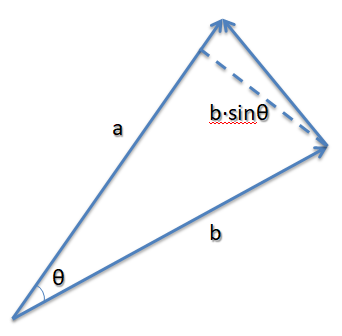所以：
\begin{aligned} \left \|\vec{a}\times\vec{b}\right \| & = \left \| \vec{a} \right \|\left \| \vec{b} \right \|\cdot sin\theta\\ & = (\left \| \vec{a} \right \|)\times (\left \| \vec{b} \right \|\cdot sin\theta)\\ & = 底 \times 高\\ & = 2\times (\frac{底 \times 高}{2})\\ & = 2\times S_{三角形} \end{aligned}
总结
向量点乘
定义
$\vec{a}\cdot\vec{b} = x_ax_b+y_ay_b+z_az_b$
几何意义与作用举例
$\vec{a}\cdot\vec{b} =\left \| \vec{a} \right \|\cdot\left \| \vec{b} \right \|cos\theta$

可以算出向量的夹角
可以直接根据此值判断两向量方向的关系：-1~ 0 ~1对应于相反 ~ 垂直 ~ 相同。

向量叉乘
定义
$\vec{a}\times\vec{b} = (y_az_b-z_ay_b,z_ax_b-x_az_b,x_ay_b-y_ax_b)$
几何意义与作用举例1
结果的向量与原先两个向量都垂直

可以用来快速算出两个向量确定的一个平面的法向量方向。

几何意义与作用举例2
$\left \|\vec{a}\times\vec{b}\right \| =\left \| \vec{a} \right \|\left \| \vec{b} \right \|sin\theta$

可以用来在已知顶点位置情况下，快速算出空间内一个三角形的面积。


展开全文数学
• 一直以来，我都记不住向量叉乘的结果，每次都要查询。其根本原因在于，我没有去研究过叉乘是如何推导出来的。于是，这次想彻底解决一下。首先要感谢维基百科，它已经把所有问题都描述...首先我们知道 ，对于向量u...


一直以来，我都记不住向量叉乘的结果，每次都要查询。其根本原因在于，我没有去研究过叉乘是如何推导出来的。于是，这次想彻底解决一下。首先要感谢维基百科，它已经把所有问题都描述清楚了。
http://en.wikipedia.org/wiki/Cross_product

而下面的文字，只是我的读书笔记，以加深自己的印象。

首先我们知道 ，对于向量u和v, u x v的结果，是得到一个既垂直于u又垂直于v的向量,假设记作n.
则有下面公式
n = u x v;
而n的方向，是由右手法则决定的。 即伸出右手，四个手指方向从u绕到v. 此时，大姆指的方向，就是n的方向。 我们通常叫做右向量。
引用一下维基百科的图来说明问题，有兴趣的兄弟可以照图比划一下。 （注：图中向量是用的a x b来表示）有了上面的知识，我们继续向下看。
我们假设向量 u,v,n分别用三个标量来表示。即
u = (Xu,Yu,Zu)
v = (Xv,Yv,Zv)
n = (Xn,Yn,Zn)
则，它们的关系为
Xn = Yu*Zv – Zu*Yv;
Yn = Zu*Xv – Xu*Zv;
Zn = Xu*Yv – Yu*Xv;
即 n = (Yu*Zv – Zu*Yv,Zu*Xv – Xu*Zv,Xu*Yv – Yu*Xv);
而为了验证n与u和v的垂直性，可以使用点乘进行
点乘法则比这个简单多了， u*v = (Xu*Xv + Yu*Yv + Zu*Zv) = dotUV;
如果两个向量垂直，则dotUV = 0;
代入验证一把
u*n = (Xu*(Yu*Zv – Zu*Yv) + Yu*(Zu*Xv – Xu*Zv) + Zu*(Xu*Yv – Yu*Xv));
= Xu*Yu*Zv – Xu*Zu*Yv + Yu*Zu*Xv – Yu*Xu*Zv + Zu*Xu*Yv – Zu*Yu*Xv;
把正负号的因式仔细比对一下，发现刚好可以低消。 结果为0.
v*n 同理可证。
于是，也验证了n与u,v垂直的特性。

如果只是为了应用的话，走到这一步就可以停下了。后面的知识，只是为了满足一下好奇心。
那我们就来看看，这个结论是怎么来的呢？ 我们接着来推导。
为了更好地推导，我们需要加入三个轴对齐的单位向量。
i,j,k.
i,j,k满足以下特点
i = j x k; j = k x i; k = i x j;
k x j = –i; i x k = –j; j x i = –k;
i x i = j x j = k x k = 0; (0是指0向量）
由此可知，i,j,k是三个相互垂直的向量。它们刚好可以构成一个坐标系。
这三个向量的特例就是 i = (1,0,0) j = (0,1,0) k = (0,0,1)。

好，那对于处于i,j,k构成的坐标系中的向量u,v我们可以如下表示
u = Xu*i + Yu*j + Zu*k;
v = Xv*i + Yv*j + Zv*k;

那么 u x v = (Xu*i + Yu*j + Zu*k) x (Xv*i + Yv*j + Zv*k)
= Xu*Xv*(ixi) + Xu*Yv*(i x j) + Xu*Zv*(i x k) + Yu*Xv*(j x i) + Yu*Yv*(j x j) + Yu*Zv*(j x k) + Zu*Xv*( k x i ) + Zu*Yv(k x j) + Zu*Zv(k x k)
由于上面的i,j,k三个向量的特点，所以，最后的结果可以简化为
u x v = (Yu*Zv – Zu*Yv)*i + (Zu*Xv – Xu*Zv)j + (Xu*Yv – Yu*Xv)k;
于是，在i,j,k构成的坐标系中。集就是上面的结果。
当i = (1,0,0) j = (0,1,0) k = (0,0,1)时，我们通常省略i,j,k的写法。最终也就得到了我们的右向量。

叉乘的意义
叉乘表示垂直于uxv的右向量。
使用的地方
可以通过叉乘，修正向量关系，从而构建坐标系。 常见的有 摄相机矩阵和TBN空间转换矩阵的构建。

叉乘的矩阵表示法。

很多书上，包括 3D游戏大师编程技巧 上面，都是用的矩阵表示法来说明叉乘。
如下。它对应的矩阵表示法为求其代数余子式，可以表示为如下有了这个，那我们合并公因式i,j,k，则可以得到矩阵表示法到此，叉乘的内容基本OK了。 值得说明的是，如果对方程组表示成矩阵不熟悉，就会感到不习惯，但是如果多多练习，我想应该是会习惯成自然吧。。。

作者：码瘾少年·麒麟子
出处：http://www.cnblogs.com/geniusalex/
蛮牛专栏：麒麟子
简介：09年入行，喜欢游戏和编程，对3D游戏和引擎尤其感兴趣。
版权声明：本文版权归作者和博客园共有，欢迎转载。转载必须保留此段声明，且在文章页面明显位置给出原文连接，否则保留追究法律责任的权利。
转载：http://www.cnblogs.com/geniusalex/archive/2013/05/09/3068158.html


展开全文• 一直以来，我都记不住向量叉乘的结果，每次都要查询。...首先我们知道 ，对于向量uv, u x v的结果，是得到一个既垂直于u又垂直于v的向量,假设记作n.则有下面公式n = u x v;而n的方向，是由右手法则决定的。 即伸...
一直以来，我都记不住向量叉乘的结果，每次都要查询。其根本原因在于，我没有去研究过叉乘是如何推导出来的。于是，这次想彻底解决一下。首先要感谢维基百科，它已经把所有问题都描述清楚了。而下面的文字，只是我的读书笔记，以加深自己的印象。首先我们知道 ，对于向量u和v, u x v的结果，是得到一个既垂直于u又垂直于v的向量,假设记作n.则有下面公式n = u x v;而n的方向，是由右手法则决定的。 即伸出右手，四个手指方向从u绕到v. 此时，大姆指的方向，就是n的方向。 我们通常叫做右向量。引用一下维基百科的图来说明问题，有兴趣的兄弟可以照图比划一下。 (注：图中向量是用的a x b来表示)有了上面的知识，我们继续向下看。我们假设向量 u,v,n分别用三个标量来表示。即u = (Xu,Yu,Zu)v = (Xv,Yv,Zv)n = (Xn,Yn,Zn)则，它们的关系为Xn = Yu*Zv – Zu*Yv;Yn = Zu*Xv – Xu*Zv;Zn = Xu*Yv – Yu*Xv;即 n = (Yu*Zv – Zu*Yv,Zu*Xv – Xu*Zv,Xu*Yv – Yu*Xv);而为了验证n与u和v的垂直性，可以使用点乘进行点乘法则比这个简单多了， u*v = (Xu*Xv + Yu*Yv + Zu*Zv) = dotUV;如果两个向量垂直，则dotUV = 0;代入验证一把u*n = (Xu*(Yu*Zv – Zu*Yv) + Yu*(Zu*Xv – Xu*Zv) + Zu*(Xu*Yv – Yu*Xv));= Xu*Yu*Zv – Xu*Zu*Yv + Yu*Zu*Xv – Yu*Xu*Zv + Zu*Xu*Yv – Zu*Yu*Xv;把正负号的因式仔细比对一下，发现刚好可以低消。 结果为0.v*n 同理可证。于是，也验证了n与u,v垂直的特性。如果只是为了应用的话，走到这一步就可以停下了。后面的知识，只是为了满足一下好奇心。那我们就来看看，这个结论是怎么来的呢？ 我们接着来推导。为了更好地推导，我们需要加入三个轴对齐的单位向量。i,j,k.i,j,k满足以下特点i = j x k; j = k x i; k = i x j;k x j = –i; i x k = –j; j x i = –k;i x i = j x j = k x k = 0; (0是指0向量)由此可知，i,j,k是三个相互垂直的向量。它们刚好可以构成一个坐标系。这三个向量的特例就是 i = (1,0,0) j = (0,1,0) k = (0,0,1)。好，那对于处于i,j,k构成的坐标系中的向量u,v我们可以如下表示u = Xu*i + Yu*j + Zu*k;v = Xv*i + Yv*j + Zv*k;那么 u x v = (Xu*i + Yu*j + Zu*k) x (Xv*i + Yv*j + Zv*k)= Xu*Xv*(ixi) + Xu*Yv*(i x j) + Xu*Zv*(i x k) + Yu*Xv*(j x i) + Yu*Yv*(j x j) + Yu*Zv*(j x k) + Zu*Xv*( k x i ) + Zu*Yv(k x j) + Zu*Zv(k x k)由于上面的i,j,k三个向量的特点，所以，最后的结果可以简化为u x v = (Yu*Zv – Zu*Yv)*i + (Zu*Xv – Xu*Zv)j + (Xu*Yv – Yu*Xv)k;于是，在i,j,k构成的坐标系中。集就是上面的结果。当i = (1,0,0) j = (0,1,0) k = (0,0,1)时，我们通常省略i,j,k的写法。最终也就得到了我们的右向量。叉乘的意义叉乘表示垂直于uxv的右向量。使用的地方可以通过叉乘，修正向量关系，从而构建坐标系。 常见的有 摄相机矩阵和TBN空间转换矩阵的构建。叉乘的矩阵表示法。很多书上，包括 3D游戏大师编程技巧 上面，都是用的矩阵表示法来说明叉乘。如下。它对应的矩阵表示法为求其代数余子式，可以表示为如下有了这个，那我们合并公因式i,j,k，则可以得到矩阵表示法到此，叉乘的内容基本OK了。 值得说明的是，如果对方程组表示成矩阵不熟悉，就会感到不习惯，但是如果多多练习，我想应该是会习惯成自然吧。。。
展开全文• Unity中点乘和叉乘点乘（API: Vector3.Dot()）点乘的计算公式点乘的几何意义用途之一：判断一个物体当前方位利用点乘求出角度叉乘（API: Vector3.Cross()）叉乘计算公式叉乘的几何意义判断物体是在左侧还是右侧 ...
Unity中点乘和叉乘点乘（API: Vector3.Dot()）点乘的计算公式点乘的几何意义用途之一：判断一个物体当前方位利用点乘求出角度叉乘（API: Vector3.Cross()）叉乘计算公式叉乘的几何意义判断物体是在左侧还是右侧
点乘（API: Vector3.Dot()）
点乘的计算公式
向量A(X1,Y1,Z1)
向量B(X2,Y2,Z2)
A•B=X1*X2+Y1*Y2+Z1*Z2
向量•向量=标量（一个数值没有方向）
点乘的几何意义
点乘可以得到一个向量在自己向量上投影的长度
如下图所示:
向量a点乘向量b就是b向量在a向量上的投影长度。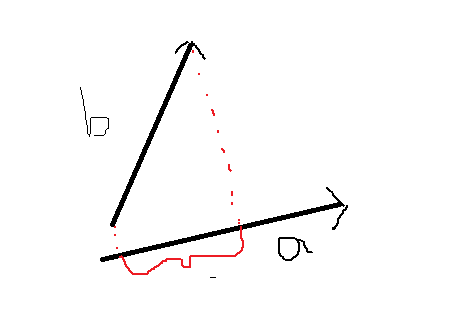意义：
点乘结果>0  两个向量夹角为锐角
点乘结果=0 两个向量夹角为直角
点乘结果<0 两个向量夹角为钝角

用途之一：判断一个物体当前方位
通过上述介绍如何判断一个物体距离自己的大致方位呢？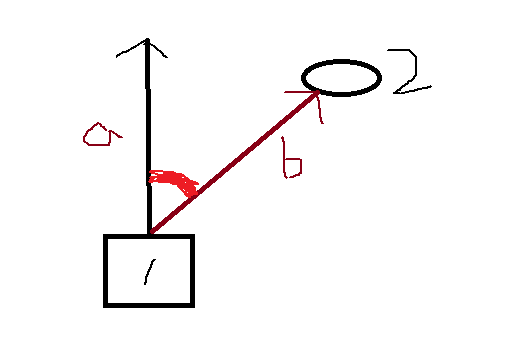假设1是用户，要求出物体2在自己的大致方位
思路：根据点乘的知识用物体1正前方的向量点乘物体2与1之间的向量，得到一个数值与0比较，如果大于0，在物体1的前面，如果等于0，在物体1的正左侧或者正右侧，如果小于0，则在物体1的后面。
Unity中点乘API:  Vector3.Dot()
代码:
public class Text1 : MonoBehaviour
{
public Transform testObj;

void Update()
{
Debug.DrawLine(this.transform.position, this.transform.forward, Color.white);
Debug.DrawRay(this.transform.position, testObj.position - this.transform.position, Color.red);

float value = Vector3.Dot(this.transform.forward, testObj.position - this.transform.position);
if (value>=0)
{
print("在我的前方");
}
else if (value<0)
{
print("在我的后方");
}
}
}

前方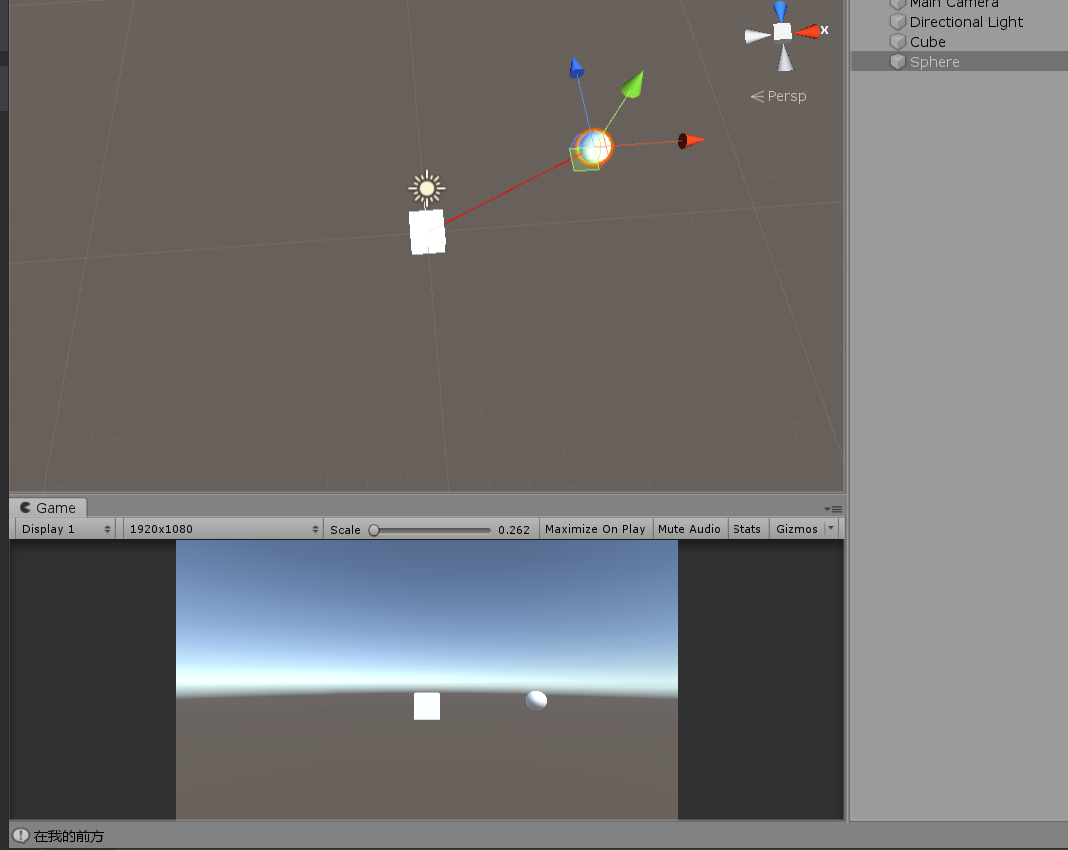后方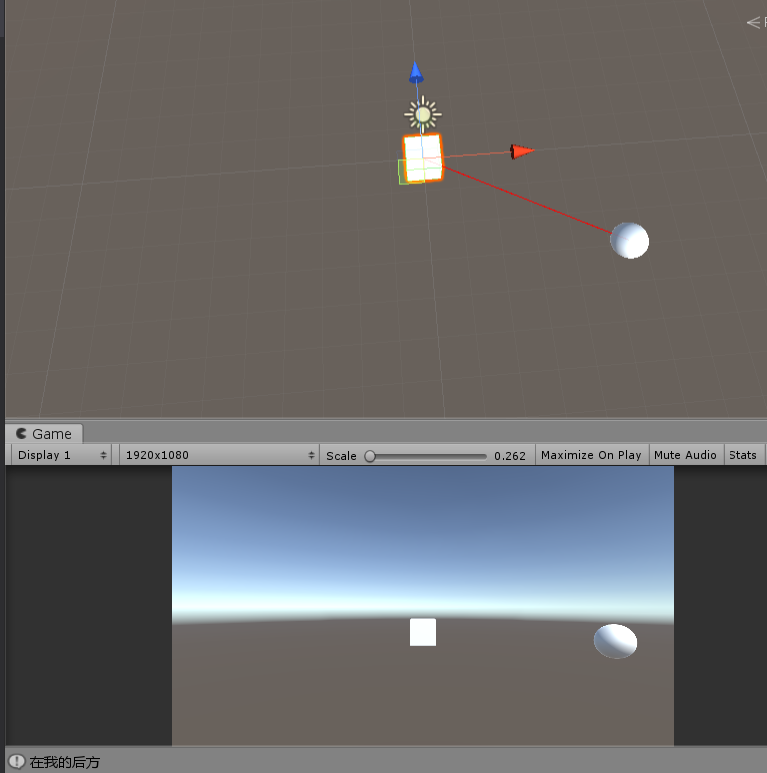利用点乘求出角度
直接求角度的API: Vector3.Angle();
推导过程：（两个向量都是单位向量）
如图所示：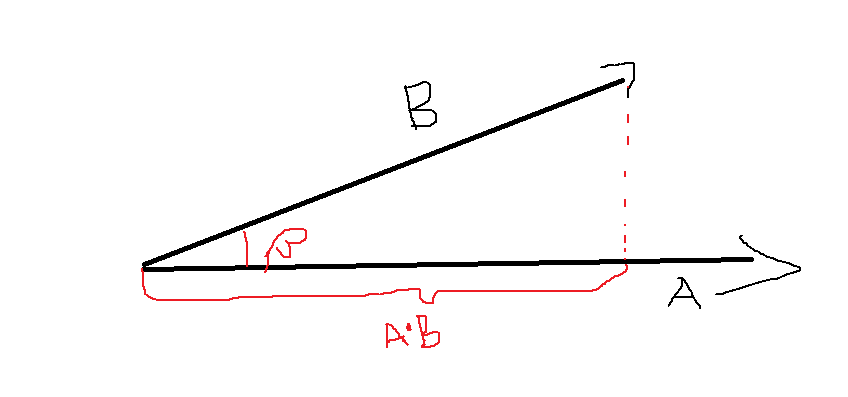Cosβ=直角边/单位向量B的模长
直角边=单位向量A•单位向量B
所以Cosβ=单位向量A•单位向量B
利用反余弦公式推出  β=Acos(单位向量A•单位向量B)  β为弧度

代码：
public class Text1 : MonoBehaviour
{
public Transform testObj;

void Update()
{
Debug.DrawLine(this.transform.position, this.transform.forward, Color.white);
Debug.DrawRay(this.transform.position, testObj.position - this.transform.position, Color.red);

float value = Vector3.Dot(this.transform.forward, (testObj.position - this.transform.position).normalized);
}
}

测试结果：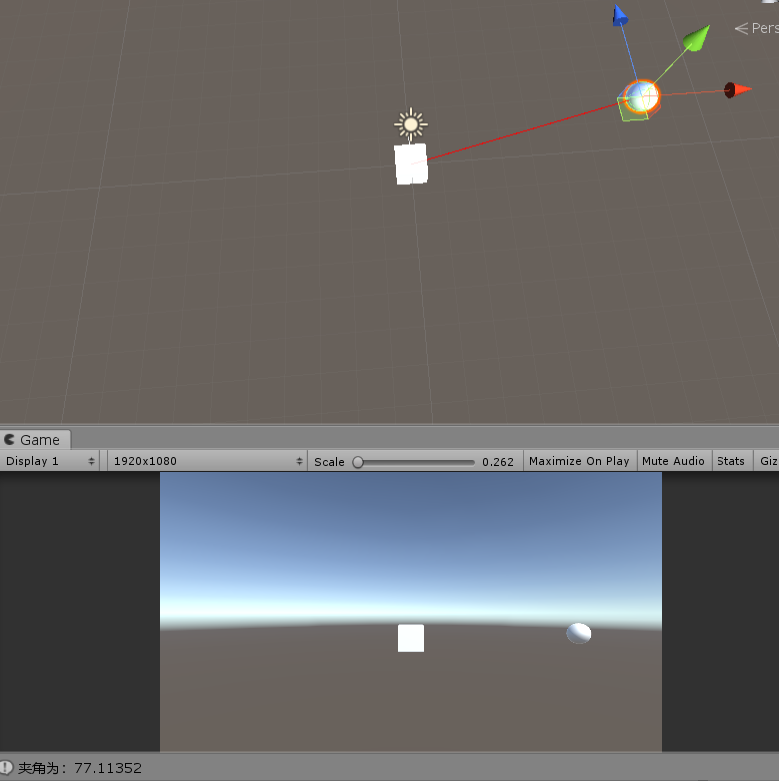叉乘（API: Vector3.Cross()）
叉乘计算公式
向量 x 向量=向量
公式:
向量A(X1,Y1,Z1)
向量B(X2,Y2,Z2)
AxB=(Y1Z2-Z1Y2,Z1X2-X1Z2,X1Y2-Y1X2)

叉乘的几何意义
AxB得到的向量同时垂直A和B
AxB向量垂直于A和B组成的平面
AxB=-(BxA)
规律：
前提条件：假设向量A和B都在XZ平面上
向量 A 叉乘 向量 B
Y大于0 证明B在A的右侧
Y小于0 证明B在A的左侧
判断物体是在左侧还是右侧
根据上述规律：
可以得出
public class Test2 : MonoBehaviour
{
public Transform transA;
public Transform transB;

void Update()
{
Vector3 v = Vector3.Cross(transA.position, transB.position);
if (v.y>0)
{
print("B在A的右侧");
}
else
{
print("B在A的左侧");
}
}
}

打印结果：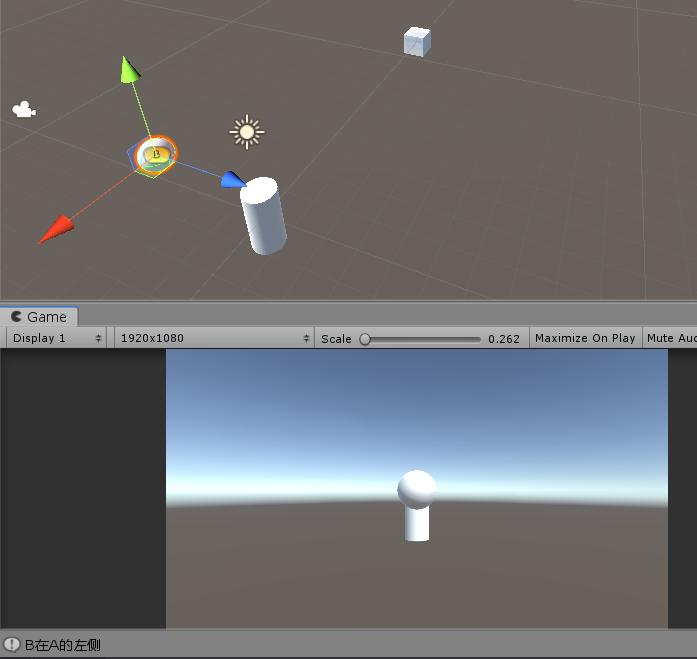`
展开全文• 菜菜的小菜在长期的工作积累中发现：基础知识的深入刨析真的很重要，尤其是在遇到一些复杂的高层次的功能块面设计的时候...小菜在接下来的计划就打算先从简单的向量和矩阵着手，先来尝试下通过自己的流程方式实...
• 为了在写作阅读时保持清晰的逻辑清醒的头脑，本文仅对四种最常见的数组乘法给出详细说明，并用一道数学题来演示向量点乘和叉乘的用法。1. 星乘(*)先声明一下：星乘这个说法，是我自己创造的，因为我实在不知道...
• 比如敌人再附近，点乘可以求出玩家面朝方向敌人方向的夹角，叉乘可以得出左转还是右转更好的转向敌人 Part 1 点乘： 数学上的 点乘为 a * b = |a| * |b| * cos(Θ) Unity中的点乘也是如此 点乘结果为 ...Unity 笔记
• 其实在高中阶段的数学，不仅仅只有代数与代数之间（函数与导数，集合与逻辑命题等）几何与几何之间（圆与圆锥曲线，平面向量与空间向量等），更多的可能是数学学科思想整个的贯穿，以及你自己强行去建立的某些联系。...
• 向量的长度现在我们已经解释了关于坐标系系统的一些东西，我们接下来可以来看看最常见的一些被用到点和向量上的操作了。在任何3D程序或者渲染器中，这些操作应该都是非常常见的。单位化一个向量如同我们前面介绍的...
• ## 向量的点乘与叉乘的几何意义

万次阅读 多人点赞 2016-11-06 21:59:35
向量的点乘与叉乘的几何意义　很惭愧，作为一名学生，向量的最基本的知识全忘了，在最近做计算机图形学实验时，需要用到向量计算时，发现自己寸步难行。只好赶快百度”预习”一下。向量的点乘:a * b公式：a * b = |a...数学
• 自己读一遍前面发现讲的太繁琐了请直接看解释2解释1 已经跑题 。。。不用看解释3 太繁琐没什么意思 但以前写的也不想删了三个解释解释1 在二维中 行列式最初是用来表示 坐标系中的图形相对于单位小正方体的缩放比例 ...
• 迫于队伍需要，蒻最近几天一直在刷计算几何的入门，做了两天，10道题，...首先，向量的两种运算，叉乘和点乘，例如向量A(X1,Y1)，B(X2,Y2)； 叉乘：A×B=（X1*Y2）-（X2*Y1）=|A|*|B|*conθ 点乘：A*B=（X1*X2）+（Y1*Y
• 自定义向量类定义和向量四则运算，包括点乘和叉乘。可以实现不规则图形面积计算。带测试例子，已自己测试通过c#
• 一直以来，我都记不住向量叉乘的结果，每次都要查询。其根本原因在于，我没有去研究过叉乘是如何推导出来的。于是，这次想彻底解决一下。...首先我们知道 ，对于向量uv, u x v的结果，是得到一个既
• 1.点乘和叉乘的定义 1.前后 Dot，返回值为正时,目标在自己的前方,反之在自己的后方 2.左右 Cross 返回值为正时,目标在自己的右方,反之在自己的左方 3.还可以用反三角函数求出值，也可以用ABS把得到的值求出绝对值...
• 面试官后来提示说从几何的角度考虑，最好不要去用方程求解的角度去想，，，大概想到了向量点乘和叉乘，但是当时没写出来。后来在网上看博客大部分也都是去解方程，稍微麻烦一些，现在把自己的思路分享一下，有错误...线性代数 算法
• Revit作为一款三维建模软件，表达的是各种结构的空间关系，二次开发中自然也包括大量的向量计算，最后发现，开发到最后都是在跟数学打交道。...两个向量之间的叉乘（结果为两个向量构成平面的法向量，遵守右c#
• ## 向量的除法-复数

千次阅读 2018-09-10 21:55:59
我们知道向量有加减法，向量有数乘、点乘和叉乘多种运算，唯独向量没有除法运算。这未免有些遗憾。我相信很多学生都想过这样的问题，向量到底有没有除法运算？ 先不说到底有还是没有，我在这里没有准备介绍中学生...
• 为了在写作阅读时保持清晰的逻辑清醒的头脑，本文仅对四种最常见的数组乘法给出详细说明，并用一道数学题来演示向量点乘和叉乘的用法。1. 星乘（*）先声明一下：星乘这个说法，是我自己创造的，因为我实在不知道...numpy 点乘
• 矩阵(Matrix)对象：线性代数有提及，此处主要是讲它在OpenGL中的用法 位置向量(Position Vector)：起点是原点的向量 ...在OpenGL中运用需要自己定义相关的类对象，当然是用已封装好的GLM更方便 GLM是Op...
• 点的法向量没有顶点法向量信息，所以要自己计算；由立体几何知识可知，一个点的法向量应该等于以这个点为顶点的所有...不共线的向量叉乘得到），顶点法向量（以这个点为顶点的全部三角形的法向量）。 接着...
• 5，向量点乘和向量叉乘 自己使用C++实现一个String类 常见功能：普通构造函数，拷贝构造函数，析构函数，运算符“+”重载，运算符“=”重载，字符串“==”重载，获取字符串长度，获取制定字符在字符串中位置 #...
• 本篇博客目的是介绍一些比较容易犯错或是... （1)inv()求逆、t()求转置、determinant()求行列式、norm()求范数、cross()求两个向量叉乘、dot()求两个向量的点乘。 二：cmake  （1) 如何生成.so库文件  在CMakeLcmake opencv 常见问题